# Methods and formulas for random effect predictions in Fit Mixed Effects Model

Select the method or formula of your choice.

## Best linear unbiased predictors (BLUP)

The BLUP are the predicted values of the random terms in the model. Recall the general form of the mixed model:
The vector that produces the BLUP estimates is:

where

For further details on the notation, go to the Methods section.

## Standard error of BLUP

The estimated standard errors are the square roots of the diagonals of this matrix:

where

For further details on the notation, go to the Methods section.

## Degrees of freedom for BLUP

The degrees of freedom for the test of theBLUP component are:

where

### Notation

TermDescription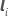a vector with value 1 at therow and 0 elsewhere with dimension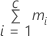Wthe asymptotic variance-covariance matrix of the variance component estimates

For further details on the notation, go to the Methods section.

## Confidence intervals for BLUP

The 100 × (1 – α)% confidence limits for the conditional expectation of thecomponent of μ given response values have the following form:

### Notation

TermDescriptiontheBLUP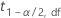the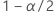percentile from the t distribution with the given degrees of freedom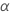1 − confidence level
dfthe degrees of freedom for theBLUP

## p-value for BLUP

The two-sided p-value for the null hypothesis that a Best Linear Unbiased Predictor (BLUP) equals 0 is:

### Notation

TermDescription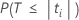The probability that under the null hypothesis T is less than the absolute value of the calculated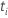. Here, T follows a t-distribution with df degrees of freedom.The t value for theBLUP.
By using this site you agree to the use of cookies for analytics and personalized content.  Read our policy